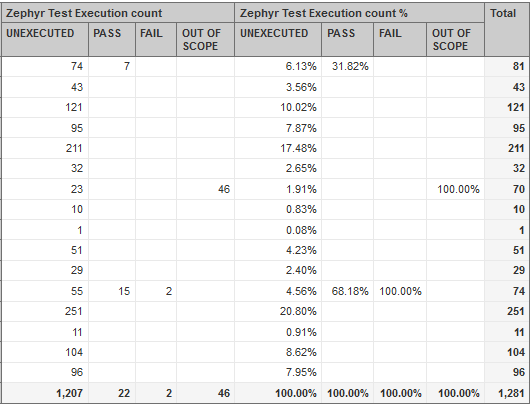# Need Sum For Rows in terms of Percentage

Hi Team ,
M looking to add values in one row, individually, and then check out the percentage.
Like if i have a total of 81 Test cases to be executed for a Prism Project ID and 74 of them are unexecuted and 7 are passed --then by using --ADD CALCULATED --> % of Total ----then is i should get 8.64 % for Pass and 91.36 % for unexecuted .

BUT

M getting sum % for columns …which m not looking for.
PSB SSIn the picture sum % is being in terms of columns …PSB 100% at end of each column .
M looking for % sum for now…like horizontal sum % and not vertical sum

eazyBI default functions Add calculated works per Column and references on all Rows data for this column.

We do not have default functions you can apply to columns. You would like to use calculated measures there. For example, if you are using Zephyr Test Status dimension on Columns and would like to get % by this dimension, you can use this formula:

``````CASE WHEN [Measures].[Zephyr Test Execution count] = 0 THEN 0
WHEN NOT IsEmpty([Measures].[Zephyr Test Execution count]) THEN
[Measures].[Zephyr Test Execution count] /
([Measures].[Zephyr Test Execution count], [Zephyr Test Status].DefaultMember)
END
``````

Daina / support@eazybi.com

1 Like